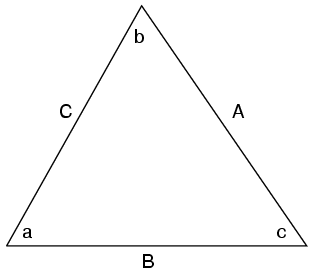# The Three Main Trigonometric Functions

Author:
Kenneth
The three main trigonometric functions are sine, cosine, and tangent. Sine is abbreviated as , cosine is abbreviated as , and tangent is abbreviated as . Don't worry if they seem intimidating at first. You'll get the hang of them.
You might have already seen these buttons on your calculator. Trigonometry is used for many other branches of math. That's why it is important for you to learn Trigonometry before venturing the harder math levels, such as Precalculus and Calculus.Sine, cosine, and tangent all have to deal with angle and side proportions. For example, if you are talking about angle a above, you can use the expressions , , and to relate angle a with the sides of the triangle.